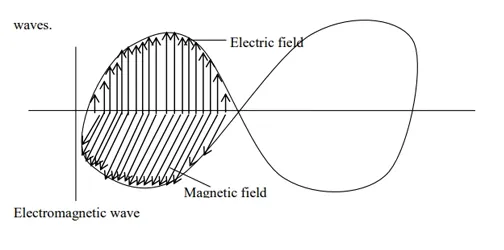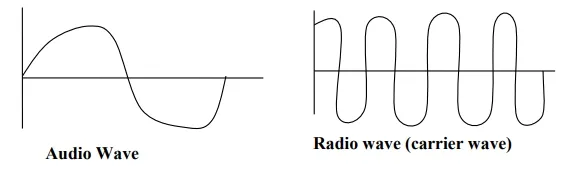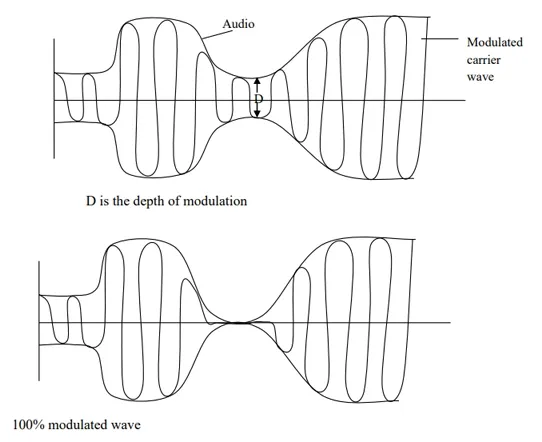## COMMUNICATION

Data communication is the process of transferring (carrying) information between two places in the form that can be handled by data communication equipment.

Message may be sent in a variety of ways   using many transmission media from the information source to its destination.

Speech enables people to communicate using sounds, with air as the transmission medium. A telephone line is an electrical line, which allows us to hold voice communication over a long distance.

### Bandwidth

Bandwidth is the range of frequencies that are available for use in a communication channel. It is expressed in hertz (Hz)

### Electromagnetic Waves

They are waves that do not require a material medium for their transmission (propagation). They travel in free space. Electromagnetic waves arise from the vibration of electric (E) and magnetic (M) fields. The combination of electric and magnetic field waves is called electromagnetic (E-M) waves.They include gamma rays, X – rays, ultraviolet rays, visible light, infrared rays, micro – waves and radio waves.

A whole range of electromagnetic wave is known as “Electromagnetic Spectrum”. The speed of electromagnetic wave in vacuum is 3.0 × 108𝑚𝑠−1

V= F⋋     OR        C= F

V= Velocity/speed of the wave in 𝑚𝑠−1

F= Frequency of the wave in Hz

= Wavelength in metre

#### Examples of Electromagnetic Waves

Numerical Example

A certain radio station is assigned a frequency of 2000KHZ. Estimate the wavelength of its radio waves. (Take C=3 × 108𝑚𝑠−1)

Solution

Frequency (f) = 2000 KHz =2 × 106Hz

C = 3 × 108𝑚𝑠−1 V = f

= C   = 3 x 108

F       2 x 106

= 150m

 Frequency Band Name Application 30KHZ – 300KHZ Low frequency (LF) AM Radio 300KHZ-3MHZ Medium Frequency (MF) AM Radio 3MHZ – 30 MHZ High Frequency (HF) AM Radio 30MHZ – 300MHZ Very High Frequency (VHF) FM Radio, TV 300MHZ – 3GHZ Ultra High Frequency (UHF) TV, Cellular, Telephony

Characteristics of Electromagnetic Waves

1.  Their wavelengths lie in the range of 1mm to a few kilometers.

2.  Have frequency range of 30KHZ – 300MHZ.

## Modulation in Digital Communication

### Modulation

Modulation is the process of varying high frequency radio waves in accordance with low frequency audio waves for transmission. Modulation is also known as frequency shifting. Radio wave is also known as carrier wave because it carriers the audio wave (human voice)#### Amplitude Modulation (AM)

Amplitude modulation is the process of varying (shifting) the amplitude of the high radio wave in accordance with the amplitude of a low audio wave.

The frequencies of the audio and radio wave remain unchanged but their amplitudes vary. AM (Modulated Envelope).

1.  It can travel long distance.

2.  Easy reception.

1.  Noise signal to noise ratio.

2.  Low bandwidth.#### Frequency Modulation (FM)

Frequency modulation is the process of varying the frequency of high radio wave in accordance with frequency of low audio wave.

1.  Better signal – to noise ratio (Less noise).

2.  Large bandwidth.

1.  Cannot be transmitted in long distance

2.  Complex in reception.

#### COST

Frequency Modulation (FM) is more expensive than Amplitude Modulation (AM) in both transmission and reception.

Signal to noise ratio is the ratio of user signal to background noise. It is usually expressed in decibels (dB).

### Demodulation

Demodulation is the process of removing carrier frequency in order for the signal to return to its original range of frequency operation.

#### Upper Sideband (USB)

Upper Sideband (USB) is the addition of signal frequency to carrier frequency.

#### Lower Sideband (LSB)

Lower Sideband (LSB) is the subtraction of signal frequency from carrier frequency.

Trial Test

1.      Metropolitan radio transmits at 4 MHZ. If the speed of the wave is 3. Calculate the length of the radio wave.

2. The wavelength of a certain radio station is 100m. If the speed of the wave is3.0 × 108𝑚𝑠−1, determine the frequency that had been assigned to the radio station.

1. V = f

= V   = 3 x 108

f        4 x 106

=7m/s

2.             f = V   = 3 x 108m/s

100m

= 3MHz